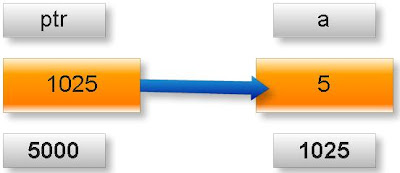## INDEX

### What is pointer in c programming?

Explain pointers in c

Pointer is a user defined data type which creates special types of variables which can hold the address of primitive data type like char, int, float, double or user defined data type like function, pointer etc. or derived data type like array, structure, union, enum.
Examples:

int *ptr;
int (*ptr)();
int (*ptr);

In c programming every variable keeps two type of value.
1. Contain of variable or value of variable.
2. Address of variable where it has stored in the memory.

(1) Meaning of following simple pointer declaration and definition:
int a=5;
int * ptr;
ptr=&a;

Explanation:

1. Name of variable : a
2. Value of variable which it keeps: 5
3. Address where it has stored in memory : 1025 (assume)

4. Name of variable : ptr
5. Value of variable which it keeps: 1025
6. Address where it has stored in memory : 5000 (assume)

Pictorial representation:Note: A variable where it will be stored in memory is decided by operating system. We cannot guess at which location a particular variable will be stored in memory.

(2) Meaning of following pointer declaration and definition:
int a=50;
int *ptr1;
int **ptr2;
ptr1=&a;
ptr2=&pt1;

Explanation:

1. Name of variable : a
2. Value of variable which it keeps: 50
3. Address where it has stored in memory : 5000 (assume)

4. Name of variable : ptr1
5. Value of variable which it keeps: 5000
6. Address where it has stored in memory : 9000 (assume)

7. Name of variable : ptr2
8. Value of variable which it keeps: 9000
9. Address where it has stored in memory : 9555 (assume)

Pictorial representation of above pointer declaration and definition:Note:
* is known as indirection operator which gives content of any variable.
& is known as reference operator which gives address where variable has stored in memory.

Cancellation rule of above two operators:
* and & operators always cancel to each other i.e.
*&p=p

But it is not right to write:
&*p=p

Simple example:

What will be output of following c program?
#include<stdio.h>
int main(){

int x=25;
int *ptr=&x; //statement one
int **temp=&ptr; //statement two
printf(“%d %d %d”.x.*ptr,**temp);
return 0;
}

Output: 25 25 25
Explanation:
As we know value of variable x is 25.

*ptr= *(&x) //from statement one
=*&x
=x //using cancellation rule
=25

**temp= **(&ptr)=*(*&ptr)=*ptr=*(&x)=*&x=x=25

Definition of pointer
Arithmetic operation with pointer
Pointer to function
Pointer to array of function
Pointer to array of string
Pointer to structure
pointer to union
Multi level pointer
Pointer to array of pointer to string
Pointer to three dimentional array
Pointer to two dimensional array
Sorting of array using pointer
Pointer to array of array
Pointer to array of union
Pointer to array of structure
Pointer to array of character
Pointer to array of integer
Complex pointer
Generic pointer
Null pointer
Wild pointer
Dangling pointer
Near pointer
Far pointer
Graphics video memory
Text video memory
Huge pointer
Memory model in C
C tutorial

Suresh said...

Good questions to learn pointers....

nidhi said...

can u xplain me y u write
int *ptr=&x;
as dis
*ptr= *(&x)

krp said...

you can write it another way
int x;
int *ptr;
ptr=&x;

in short you can write

int x;
int *ptr=&x;

that's all

heena said...

**temp= **(&ptr)=*(*&ptr)=*ptr=*(&x)=*&x=x=25
whats the meaning of this line

Afiz S said...

@Heena:
**temp= **(&ptr)=*(*&ptr)=*ptr=*(&x)=*&x=x=25

let me divide this into small pieces. left to right
x=25//
*&x=x // x=25
*&x=25//
x=25 (*and& will cancellation rule)
jut like that finally you will get

**temp = **(&ptr)=25 (*and& will canceled)
**temp=*ptr=25
**temp=25; (temp is pointer to another pointer variable called ptr)

**temp : is content of x variable

Satheesh said...

nice work.............thanks

AVINASH SINGH said...

c programming language is simple and the best language............

u can solve any problem here...
but u should knowledge about "c"

tarun trivedi said...

niceeeeeee.......

mohit said...

This comment has been removed by the author.

mohit said...

when i run the program in c
int *ptr;
ptr=new int;
*ptr=5;
cout<<(*&ptr)<<endl;
cout<<ptr<<endl;
cout<<(*ptr)<<endl;
cout<<(&*ptr)<<endl;
delete ptr;
It gives the output as
0x8d20008
0x8d20008
5
0x8d20008
but as in this tutorial it is written that it is not right to write &*p=p;
but the above result shows that its right???

Sandwich Enthusiast said...

Mohit you are mixing dialects of C...the snippet you ionserted is C++, and pointer notation in C++ isn't the same as C

sumaya zama Syed said...

Thank u soooooooooooooo much for helping me understand the concept of pointers & i hav a small doubt that is,is this a formula for pointer"a=5;ptr=&a"right......?plz send me rply

bimal shah said...

Thanks a lot for such an excellent clear concept. Really,sir....u r the master...sir i have small doubt in line[printf(“%d %d %d”.x.*ptr,**temp); ] is it .x. Or ,x, i.e [ printf(“%d %d %d”,x,*ptr,**temp);